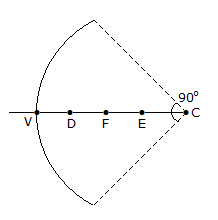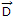Courses

# Test: Electromagnetic Field Theory - 4

## 25 Questions MCQ Test Electromagnetic Theory | Test: Electromagnetic Field Theory - 4

Description
This mock test of Test: Electromagnetic Field Theory - 4 for Electrical Engineering (EE) helps you for every Electrical Engineering (EE) entrance exam. This contains 25 Multiple Choice Questions for Electrical Engineering (EE) Test: Electromagnetic Field Theory - 4 (mcq) to study with solutions a complete question bank. The solved questions answers in this Test: Electromagnetic Field Theory - 4 quiz give you a good mix of easy questions and tough questions. Electrical Engineering (EE) students definitely take this Test: Electromagnetic Field Theory - 4 exercise for a better result in the exam. You can find other Test: Electromagnetic Field Theory - 4 extra questions, long questions & short questions for Electrical Engineering (EE) on EduRev as well by searching above.
QUESTION: 1

Solution:
QUESTION: 2

Solution:
QUESTION: 3

### To couple a coaxial line to a parallel to wire line it is best to use a

Solution:
QUESTION: 4

The shunt admittance of a transmission line is given by

Solution:
QUESTION: 5

A dipole antenna was radiating with some excitation in free space radiating a certain amount of the power. If this antenna is immersed in a lake where water is non-dissipative but has a dielectric constant of 81, the radiated power with the same excitation will

Solution:
QUESTION: 6

If short-circuited and open circuited impedances are 5 ohms and 20 ohms, then characteristic impedance is

Solution:
QUESTION: 7

Electric lines of force do not cross each other because

Solution:
QUESTION: 8

A person with a receiver is 5 km away from the transmitter. What is the distance that this person must move further to detect at 3 dB decrease in signal strength?

Solution:
QUESTION: 9

Solution:
QUESTION: 10

SHF range is

Solution:
QUESTION: 11

For an ideal antenna, numerical value of directivity must be

Solution:
QUESTION: 12

The force in a magnetic field is given by F = qvB

Solution:
QUESTION: 13

An electromagnetic wave is incident normally on a dielectric boundary. It is

Solution:
QUESTION: 14

According to maximum power transfer theorem, if the load is inductive, the source should be

Solution:
QUESTION: 15

According to Siegel and Labus, antenna can be treated as

Solution:
QUESTION: 16

Given figure shows a 90º sector of spherical reflector with vertex 'V', centre of curvature 'C' and equal distances VD, DF, FE and EC. When a beam of EM wave is incident parallel to the axis, all the energy will pass through the regionSolution:
QUESTION: 17

Self impedance is

Solution:
QUESTION: 18

The value of susceptibility in case of vacuum is taken as

Solution:
QUESTION: 19

Equipotential surfaces about a point charge are in which one of the following forms?

Solution:
QUESTION: 20

__________ will have the least value of relative permeability.

Solution:
QUESTION: 21

The relative permeability is taken as unity for which of the following?

Solution:
QUESTION: 22

The disturbance from adjacent power lines is known as

Solution:
QUESTION: 23

Which of the following term applies to troposcatter propagation?

Solution:
QUESTION: 24

Magnesium is alloyed with conducting materials for antenna primarily with the purpose of

Solution:
QUESTION: 25

Two co-axial cylindrical sheets of charge are present in free space fs = 5 c/m2 at r = 2 m and rs = -2 c/m2 at r = 4 m, The displacement flux densityat r = 3 m is

Solution: